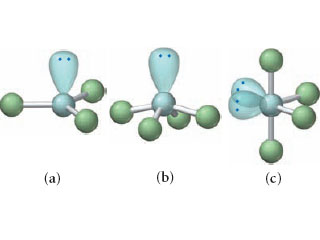# Problem: Each of the following ball-and-stick models shows wrong electron and molecular geometry of a generic molecule.Provide the correct molecular geometry for (a), given the number of lone pairs and bonding groups on the central atom.

###### FREE Expert Solution
83% (163 ratings)
###### Problem Details

Each of the following ball-and-stick models shows wrong electron and molecular geometry of a generic molecule.Provide the correct molecular geometry for (a), given the number of lone pairs and bonding groups on the central atom.

Frequently Asked Questions

What scientific concept do you need to know in order to solve this problem?

Our tutors have indicated that to solve this problem you will need to apply the Electron Geometry concept. You can view video lessons to learn Electron Geometry. Or if you need more Electron Geometry practice, you can also practice Electron Geometry practice problems.